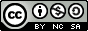## Tuesday, March 18, 2008

### Using Unpack To Convert Binary Values To Decimal

Hey There,

Today's post may seem like a step back for regular readers, but I like to keep this blog accessible, so I've decided to revisited the Perl unpack function to demonstrate how it can be used in one of its simplest forms on either Linux or Unix. A more advanced example can be found in our previous post on taking a look inside the wtmpx file.

The Perl script we're presenting today only consists of one simple unpack function surrounded by a whole bunch of processing code. If certain parts were modularized, this would make a decent simple function in a greater calculator/conversion script.

Notice that when we use the unpack value to convert a binary number, say:

11101110

to the decimal number:

238

all we're really doing is feeding the value (11101110) to the unpack function and telling it to convert the input using a standard template ("C" in this case - which stands for an unsigned character - octet - value). You'll not that we've actually put "C*" to account for an infinitely variable number of unsigned octet character values. If we didn't do this (Just put "C" instead), the unpack function would only process the first character the script fed it, so our original input:

11101110

would translate to this:

1

So, as you can see, in this simple function - unpack("C*", \$binary) - the definition of the predefined template value C can make a huge amount of difference. It can also allow you to be as specific as you need to be. For instance - unpack("CC", \$binary) - would produce:

3

from the same input. This can also be written as - unpack("C2", \$binary) - which will save you a lot of typing if you want to match exactly 245 C's ;)

Hope you find this script useful and that it helps you to get more into Perl's pack and unpack functions. Once you get past the seeming incomprehensibility of the general statements, it all makes sense :)

Cheers,`#!/usr/bin/perl## bd - convert binary numbers to decimal## 2008 - Mike Golvach - eggi@comcast.net## Creative Commons Attribution-Noncommercial-Share Alike 3.0 United States License#print "Binary Number:\t";chomp (\$binary=<stdin>);@bitvalue = unpack("C*", \$binary);\$bitvalue = @bitvalue;\$substring = \$bitvalue - 1;while (\$substring ne -1) { \$number = substr(\$binary, \$substring, 1); \$binarray[\$substring] = \$number; --\$substring;}@decarray = reverse(@binarray);\$exponent = 0;foreach \$subscript (@decarray) { if (\$subscript == 1) { \$subscript = 2 ** \$exponent; } elsif (\$subscript == 0) {  \$subscript = 0; } else {  print "Are you sure you know what a Binary Number is?\nMaybe you shouldn't be using this...\n";  exit; } \$exponent++;}\$decimal = "0";foreach \$integer (@decarray) { \$decimal += \$integer;}print "Decimal Value:\t\$decimal\n";exit;`